# Graphing linear functions using table of values

## How to graph a linear equation

A linear equation is an equation that has two variables that when graphed gives us a straight line. You probably know how to read a linear equation graph, but how do we go about plotting out a linear equation onto a coordinate plane? It turns out it's actually not that hard! The first step is learning how to do a table of values.

## What is a table of values

When we have to graph out a linear equation, you'll start by creating a table of values. A table of values, as its name suggests, is a graphical way to determine the values that will be used to create your graph. It's a place for you to jot down the answers you get when you find the value of x and y. You'll need to use at least 2 sets of points to graph out a linear equation, but you will usually do more in a table of values.

A table of values has two columns. One column lists the x values, and one column lists the corresponding y values. Let's move on to finding out how this table can be used to help us graph out linear equations.

## Graphing linear equations using a table of values

When you've got a blank table of values and a linear equation that you want to graph out, you can take any x value on the x axis of your choosing to start off your table. Let's say you take the number 3. Substitute that into the linear equation to see what you'll get when you solve for y on the y axis. Let's pretend that you get -5. Jot down both of these numbers into your table, with 3 on the column with x values and -5 on the column for y values.

Choose another x value you want to work with and solve for y again. Repeat as many times as you wish to work out sets of values to put into your table. When you've got an amount you're happy with or that your question asks for, you can then take those x and y values and plot them out as coordinates on a graph to graph points on a linear equation!

## Example problems

Question 1:

Graph following function using a table of values

y = 3x - 1

Solution:

First, create a table of values for the equation. Use x to solve for y. You may use different values, but your graph will be the same no matter what values you use.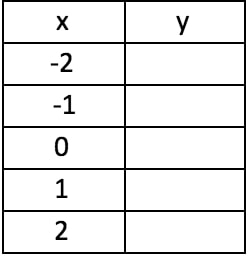Table of values of x

For x = -2

y = 3(-2) - 1

y = -6 - 1

y = -7

For x = -1

y = 3(-1) - 1

y = -3 - 1

y = -4

For x = 0

y = 3(0) - 1

y = 0 - 1

y = -1

For x = 1

y = 3(1) - 1

y = 3 - 1

y = 2

For x = 2

y = 3(2) - 1

y = 6 - 1

y = 5

Now, we have a complete table of values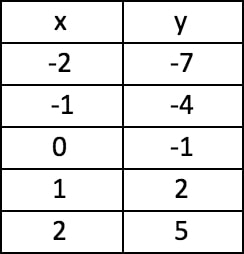A completed table of x and y values

We can now plot the ordered pairs onto a grid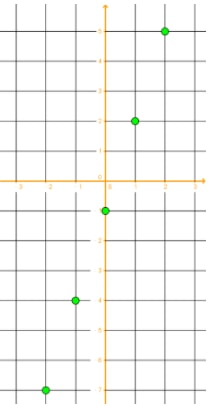Data plotting

Then, connect the points using a straight line, and we are done!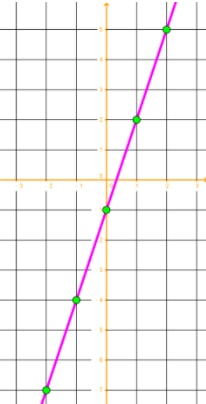Data linking in a straight line

Question 2:

Graph the following function using a table of values:

2x + 4y = 8

Solution:

First, we want to simplify the equation

2x + 4y = 8

x + 2y = 4

2y = -x + 4

y = $\frac{-1}{2}$x + 2

Now we've got an equation in slope-intercept form.

Then, create a table of values for the equation. Use x to solve for y.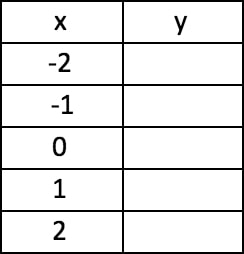Table of x solve for y

For x = -2

y = $\frac{-1}{2}$(-2) + 2

y = 1 + 2

y = 3

For x = -1

y = $\frac{-1}{2}$(-1) + 2

y = 0.5 + 2

y = 2.5

For x = 0

y = $\frac{-1}{2}$(0) + 2

y = 0 + 2

y = 2

For x = 1

y = $\frac{-1}{2}$(1) + 2

y = -0.5 + 2

y = 1.5

For x = 2

y = $\frac{-1}{2}$(2) + 2

y = -1 + 2

y = 1

Now, we have a complete table of values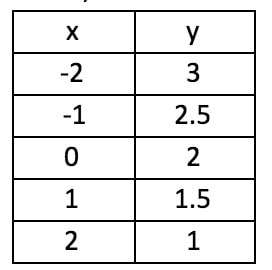Table of completed data

We can plot the ordered pairs onto a grid.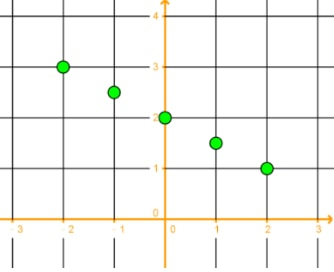Coordinates plotted using data table

Then, connect the points using a straight line, and we are done!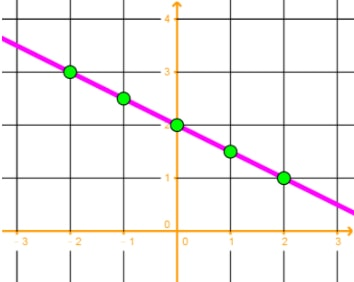Link data together with straight line

Want to check your answer to see how accurate your graph is? Here's an online graphing calculator for your reference.

Next up, learn more about table of values, how to graph linear inequalities in two variables, and how to graph systems of linear inequalities. You can also start learning an introduction to functions and how to identify them.

### Graphing linear functions using table of values

In order to graph linear function, we can make use of the table of values to map out the corresponding values of x and y for the given line. An equation is essentially a representation of the relationship of x and y values for any line. A table of values is basically a table which lists the values of y, given the x values, for the given line.

#### Lessons

• 1.
Graph following functions using the table of values
a)
y = 3x -1

b)
2x + 4y = 8